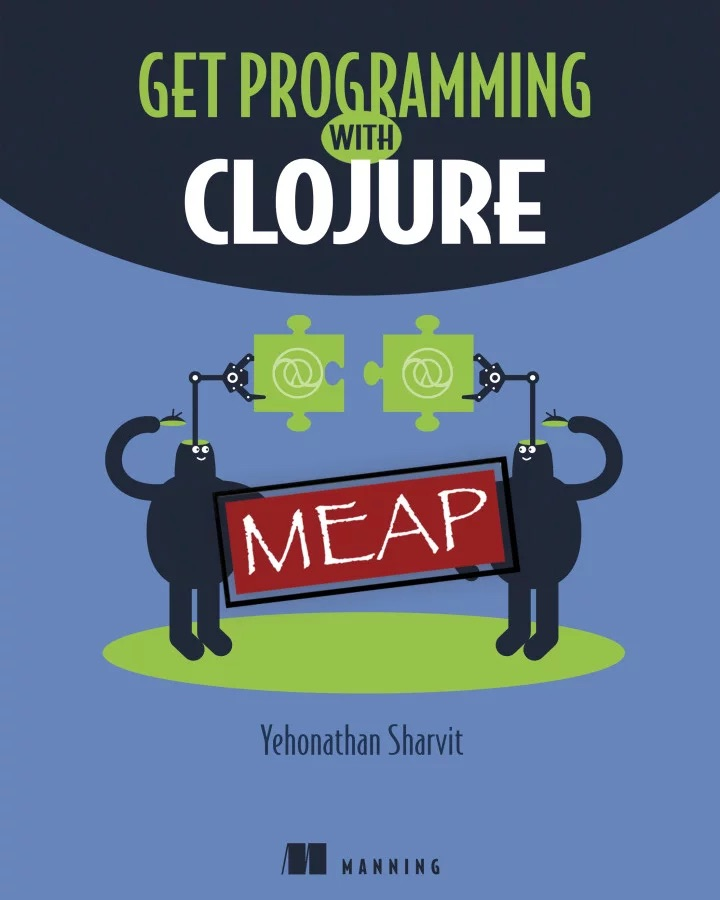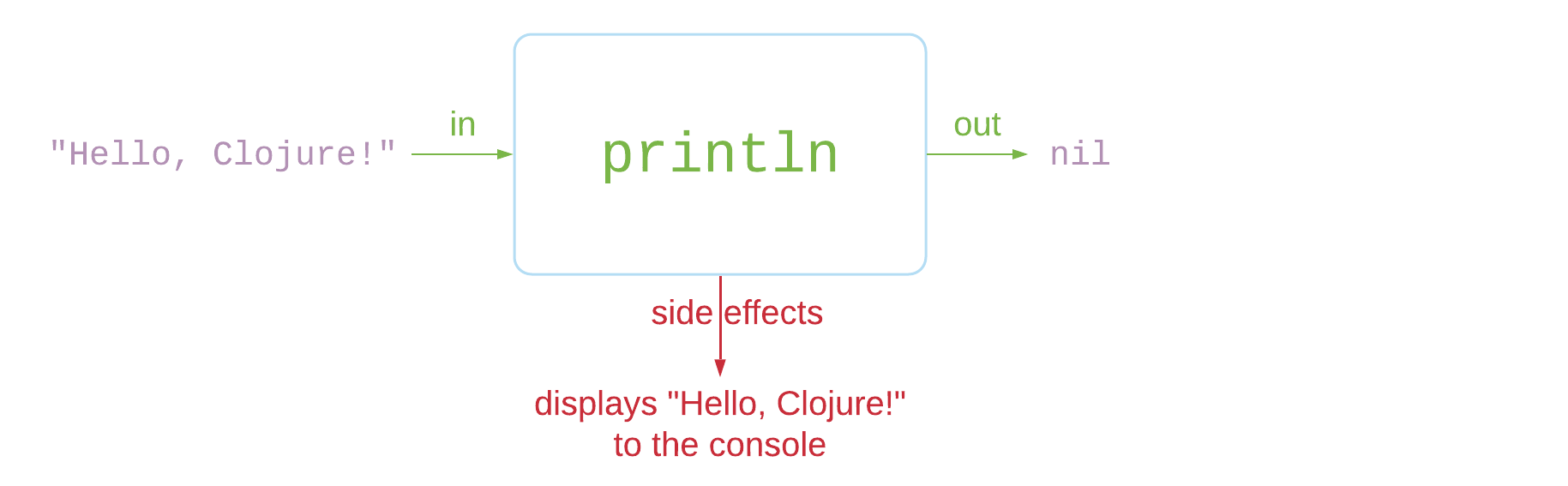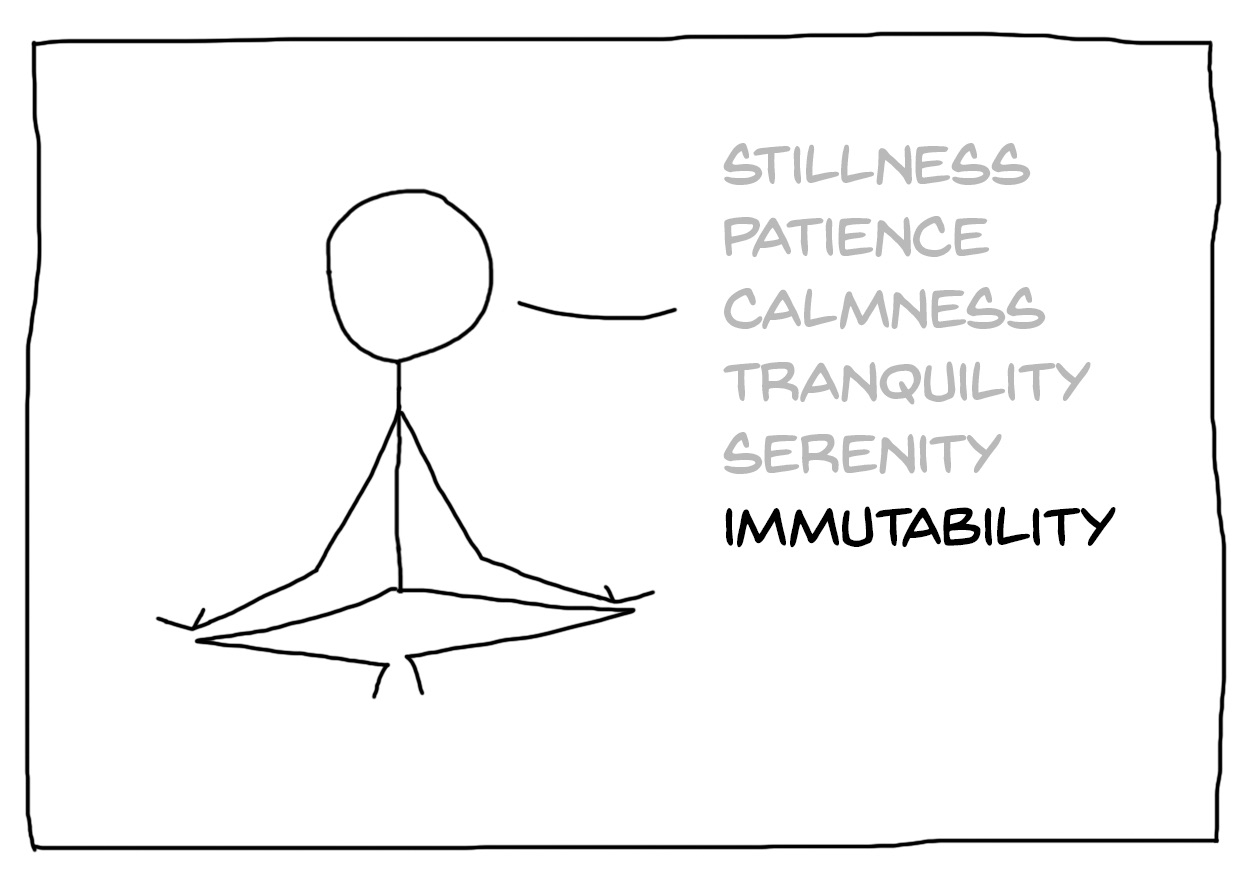Interactive SlidesYehonathan Sharvit

```        ```
(str (js/Date.))
```
```

## Who am I?

• A pragmatic theorist

• A freak of interactivity

• Author of Klipse: a client side code evaluator pluggable on any web page

• Running a small consulting company

• Sparking the joy of Clojure at Cycognito, Audyx and NextjournalYehonathan Sharvit @viebel

## Begin with the End in Mind

1. You are motivated to learn Clojure

2. You look differently at your existing Software knowledge

3. You feel you had a fun time

You want to read my book on Clojure 💸## Three+One wishes

1. I wish I could express myself clearly

2. I wish I could code in a simple way

3. I wish I could write (and read) code that is predictable

4. I wish I could get more wishes## Power of expressions## The lack of power of expressions

We cannot assign the result of an `if` or a `switch` statement to a variable.

``var a = if (true) { 5 } else {42};``
 We cannot comment out an expression. ``````function foo() { // if (true) { // return 5; // } else { // return 42; // } return 7; }`````` The syntax is not uniform: operator, statements, function calls, function definition, classes…​ ``````function foo(z) { return z + Math.sqrt(z); }``````

## The power of expressions

 Every part of the language is an expression, with the same structure
 arithmetic operation ``(+ 1 2)`` boolean operation ``(and true false)`` local bindings ``````(let [a 42] (+ a 5))`````` variable assignment ``(def my-num 42)`` `if` expression ``(if my-num "happy" "sad")`` function call ``(inc 42)``

## The power of expressions - Javascript vs. Clojure

 Javascript Clojure ``foo(1, 2)`` ``(foo 1 2)`` ``2 + 3`` ``(+ 2 3)`` ``````if (x > 2) { return 3; } else { return 5; }`````` ``````(if (> x 2) 3 5)`````` ``var myNum = 42`` ``(def my-num 42)``

## The power of expressions - the rules

1. An expression is enclosed into opening and closing parenthesis: `()`, `[]` or `{}`

2. First element inside the parentheses is the name of the expression: `def`, `if`, `+`…​

3. Other elements inside the parentheses are the arguments to be passed to the expression, either a primitive or an expression

4. When one of the arguments is itself an expression, it follows the same syntactic rules (recursively)

``````(defn foo [x y]
(if (= x (+ y y))
(* x y)
(inc x)))
(foo 42 20)``````

## The power of expressions - if expressions

We can assign the result of an `if` expression into a variable

``````(def my-mood (if my-num "happy" "sad"))
my-mood``````

## The power of expressions - comments

We can comment out a single expression out of a big piece of code

``````(defn print-moods [num]
(println "happy")
#_(if (= num 42)
(println "furious")
(println "angry"))

(print-moods 42)``````

## The power of expressions - macros

We can write code that receives source code and genenerates another code.

Challenge 1

Write a function that receives a `name` and prints to the console
Hello <name>!

You may use the language of your choice.

## Hello Javascript

``````function hello(name) {
console.log(`Hello, \${name}!`);
}
hello('Javascript');``````

Thoughts 🤔

1. Can you write a unit test?

2. Can you compose?

3. Can you cache?

## Hello Clojure

We are going to separate:

• Computation (concatenation of `"Hello"` and `name`)

• Side effects (print to the console)

## Hello Clojure

We create a function named `hello-str` that receives `name`
and "computes" the concatenation of `"Hello"` and `name`

``````(defn hello-str [name]
(str "Hello, " name "!"))``````

The `hello` function that does the side effect (print) uses `hello-str`:

``````(defn hello [name]
(println (hello-str name)))``````
``(hello "Clojure")``

## Pure functions vs. side effects

A pure functionA function with side effects## Key idea: separate side effects from computation1. Testable

2. Composable

3. Cachable

## Testable

A trivial test case

``````(deftest test-hello-clojure
(is (= (hello-str "Clojure")
"Hello, Clojure!")))``````

Check

``(test-hello-clojure)``

🤔 Can you test the function you wrote to solve the challenge?

## Composable

We can use our functions as building blocks 🧱 for other functions.

 We compose as we wish ``````(defn hello-cap [name] (upper-case (hello-str name)))``````
 It returns a composed string ``(hello-cap "Clojure")``
 We print it, later ``(println (hello-cap "Clojure"))``

🤔 Can you compose the function you wrote without modifying its code?

## Hello worlds

Handle sequences

Let’s handle sequence of names

``(map hello-str ["Clojure" "Python" "Javascript" "Java"])``
 Let’s combine the elements of the sequence, into a string ``````(defn multiple-hellos [names] (join " " (map hello names)))``````

We print, later

``(println (multiple-hellos ["Clojure" "Python" "Javascript" "Java"]))``

## Cachable

 Imagine that string concatenation were a heavy operation. ``````(defn hello-print [name] (println (str "Hello, " name "!")))``````

We would like to cache the function
Next time we call it, it returns the result immediately

 🤪 It makes no sense to cache a function with side effects! ``````(def memoized-print (memoize println)) (memoized-print "Hello!") (memoized-print "Hello!") (memoized-print "Good bye!")``````

## Interlude: A brief history of Functional Programming## 1930: Lambda Calculus

• Alonzo Church discovers the λ-calculus

• Everything is an anonymous function with a single argument

• No names in the language - only function argument

• Even numbers are expressed as functions

• 0 := λfλx.x

• 1 := λfλx.fx

• 2 := λfλx.f(fx)

## 1958: LISP

• John McCarthy invents LISP

• It is the 1st FP language

• The data is expressed as S-Expressions: `(1 2 3)`, `(a b (c d))` etc…​

• The code is made of S-Expressions: `(+ 1 2 3)`, `(if true 1 42)` etc…​

## 1995: Javascript the language of the browser

• Brendan Eich is recruited by Netscape to do "scheme in the browser"

• Eventually, he invents Javascript

• Functions are 1st class citizens

• JSON data format is similar to S-Expressions

## 2000s: Clojure

• 2007 - Rich Hickey invents Clojure - A practical dialect of LISP on top of JVM

• 2011 - ClojureScript - Clojure rocks, Javascript Reaches!

• 2013 - Facebook creates react.js - A functional javascript frontend framework

• 2015 - Dan Abramov invents redux - A javascript library that imposes FP constraints on a frontend app

## Dealing with Data is valuable## The value of values

Challenge 2

Read carefuly the following piece of code and tell me what is the value of x?

You may convert the code to the language of your choice.

``````const x = 42;
calcSomethingUseful(x);
//x``````

## The value of values

Challenge 3

Read carefuly the following piece of code and tell me what is the value of y?

You may convert the code to the language of your choice.

``````const arr = [5, 99, 1230];
const z = calcVeryUseful(arr);
const y = arr.length;
//y``````

## Welcome immutability## What is immutability?

Immutability means that data collections are treated like values.
Values never change!

 In the realm of numbers ``````(def my-num 42) (inc my-num) #_my-num`````` In the realm of strings ``````(def my-str "Hello") (str my-str " Clojure!") #_my-str`````` In the realm of vectors ``````(def my-vec [42 400 532]) (assoc my-vec 1 54) #_my-vec`````` In the realm of maps ``````(def my-map {:name "Franck"}) (assoc my-map :metadata 12321321) #_my-map``````

## The value of immutability1. Code is predictable

2. No "by reference" or "by value" headache

3. Equality check is fast

4. Concurrency friendly wihtout locks

## Beyond immutability## Atoms

 ``(def my-state (atom {}))`` ``(swap! my-state assoc :money 1000)``

 ``@my-state`` ``my-state``

## Extend the language with macros

1. It would be cool to write asynchronous code that looks synchronous

2. It would be fun to add debugging capabilities

3. It would be productive to write code that disappears on production

4. It would be nice to pass data to data manipulation functions sequentially

## Custom debugging

``````(defn complex-stuff [x & forms]
(loop [x x forms forms]
(if forms
(let [form (dbg (first forms))
threaded (list (first form) (first (next form)) x)]
x)))``````

``(complex-stuff 10 [1 2] [4 5])``

 Wrapping functions become hard to read ``````(reduce + (filter odd? (map inc (range 100))))`````` Use pipelines ``````(->> (range 100) (map inc ) (filter odd? ) (reduce + ))``````

It’s a macro

``````(macroexpand '(->>
(range 100)
(map inc)
(filter odd?)
(reduce +)))``````

## Macros Internals## Macros Internals

``````(defn transform [x & forms]
(loop [x x forms forms]
(if forms
(let [form (first forms)
threaded (list (first form) (first (next form)) x)]
x)))``````
``````(transform '(range 100)
'(map inc)
'(filter odd?)
'(reduce +))``````

## Wishes come true1. I wish I could express myself clearly
The power of expressions

2. I wish I could code in a simple way
Pure functions

3. I wish I could write (and read) code that is predictable
Immutability

4. I wish I could get more wishes
Macros

## Questions